ISIJ International
Online ISSN : 1347-5460
Print ISSN : 0915-1559
ISSN-L : 0915-1559
Regular Article
Analysis of Two-Phase Flow and Bubbles Behavior in a Continuous Casting Mold Using a Mathematical Model Considering the Interaction of Bubbles
Tao ZhangZhiguo LuoHeng ZhouBing NiZongshu Zou
Author information
JOURNALS OPEN ACCESS FULL-TEXT HTML

2016 Volume 56 Issue 1 Pages 116-125

Details
Abstract

A new mathematical model considering the process of bubbles interaction has been developed to simulate the transient fluid flow, dispersed bubbles motion and transport process in the slab continuous casting mold. Rather than prescribing a constant bubbles size in previous work, this model allow us to calculate the constantly changed daughter particles size distribution after the process of bubbles collision and breakup. In this paper, using the new model to study the effect of some parameters such as gas flow, casting speed, and the depth of submerged entry nozzle (SEN) on the fluid flow pattern, the gas volume fraction, the distribution of bubbles and so on. The predictions of gas bubble distribution and fluid flow pattern are in good agreement with the water model experimental observations. Meanwhile, the model has successfully reproduced many known phenomena and other new predictions, including the process of bubbles collision and breakup. The simulation results show that the important factors that influence the size and quantity distribution range of bubbles are casting speed and argon gas flow rate and depth of SEN. Through the statistical analysis of bubbles behavior, the effects of blowing argon on porosity defects under different operating conditions can be obtained.

1. Introduction

In continuous casting process, the mold is the final step in clean molten steel control. The introduction of argon gas injection technology make analysis of discrete phase transport to be a hot topic recently. The bubbles injection plays an important role in affecting the floatation, removal of inclusions, slag entrapment prevention, helping prevent nozzle clogging, enhancing mixing, and preventing air from the submerged entry nozzle (SEN), slab quality improvement. However the entrapment of argon bubble near the advancing solidified shell causes lots of internal defects to the slab. Getting the optimum operating conditions in the continuous casting mold is important due to their effect on the various phenomena related to the quality of the casting steel product, such as particles entrapment, transient fluctuations at the top surface, the slag formation, the transport and dissipation of superheat and so on. Therefore, it is essential to make the research on the two-phase flow of molten steel and argon bubbles in the continuous casting mold which is regarded as a key factor that determines the quality of final products.

Many research1,2,3,4,5,6,7,8,9,10,11,12,13) works have been developed to study the two-phase flow and tracked the trajectories of bubbles inside the liquid pool using a Lagrangian approach in the continuous casting process. C. Pfeiler et al.14) compared the two different methods to investigate the transport of the gas bubbles in the SEN and melt pool of steel continuous caster considering the interaction between the continuous and the dispersed. Lei and Zhang15) developed a numerical simulation model, in which level set method and modified heat transfer equation is applied to simulate the dynamic evolution of gas-liquid interface and solidification process. Thomas et al.16,17) developed a particle-capture model based on local force balances implemented into computational models of turbulent fluid flow and particle transport. Zhang and Yang18) presented two approaches to predict the particle entrapment in the full length of a billet caster. The results showed the calculated inclusion distribution in the billet by the full solidification approach agreed with the industrial measurement better than the sink term approach. Esaka et al.19) investigated the interaction between gas bubbles and solidified shell using in-situ observation. They found that entrapment of gas bubbles increases with the roughness of the solid/liquid interface. Kubo et al.20) have investigated the influence of argon gas on molten steel flow, different multiphase models were carried out for several casting speeds. Liu and Li21) developed an EELE simulation scheme to calculate the two-phase flow of argon gas and molten steel in slab continuous casting mold. In these previous works, the change of bubbles size in the process of collision and breakup in the molten steel flow was neglected, so these results only applied to distribution and transport of the constant size bubbles.

Although some papers22,23,24) have researched the theoretical model for bubble breakup and coalesce in turbulent flow. These models only were applied to the bubble columns, and that were in the perspective of statistics. However, in process of the continuous casting, very few mathematical simulation models had considered the influence of bubbles interaction on the motion of gas bubbles and the two-phase flow structure Therefore, this work developed an innovative methods to match the real processes and understand the effect of different operating conditions on the behavior of two-phase flow. Rather than prescribing a constant bubbles size in previous work, this model allow us to calculate the constantly changed daughter particles size distribution after the process of bubbles collision and breakup. Our model of bubbles interaction was established using the newly-developed codes and we used the similar discrete element method in combination with computer fluid dynamics in this paper. The first purpose of current work is to develop a new mathematical model to study the two-phase flow of molten steel and argon gas. The second is to apply this model to investigate the transport and distribution of gas bubbles, considering the effect of gas flow rate, casting speed, depth of SEN and gas bubbles size. The equations are solved with the commercial package FLUENT, with the help of extensive user defined subroutines developed by authors.

2. Mathematical Model Formulation

2.1. Interaction of Bubbles Model

2.1.1. Phenomenological Simplifications

In turbulent dispersion systems, the fluid dynamics and bubbles interaction process are complex. Hence, in order to develop the interaction model, certain simplifications are necessary.

1. Only the binary breakage of fluid particles in a turbulent dispersion is considered. As is well known, a bubble break into two or more bubbles with equal or unequal volumes, depending on the breakage type. The simplification of binary breakage has been used by many authors.25,26,27) The recent experimental results of Hesketh et al.26) support the assumption. They found that all bubbles breakage events were binary in turbulent pipeline flows. In the present model, the assumption of binary breakage is also used.

2. The breakup closure is assumed to be equal-sized. For the binary breakage, a dimensionless variable describing the sizes of daughter bubbles (the breakage volume fraction) can be defined as:

 f BV = V 1 V = d 1 3 d 3 = d 1 3 d 1 3 + d 2 3 (1)
where d1 and d2 are diameters of the daughter particles in the binary breakage of a parent bubble with diameter d. This model is based on the turbulence stress and surface tension force balance, so the equal-sized breakup occurred most likely. In this paper, fBV=0.5 means equal binary breakup.

3. Bubbles collision only depend on the distance between two bubbles. Many researchers and scholars proposed the collision frequency of bubbles in macro statistical point of view.28) However, this paper can judge how many bubbles are contacted, and then calculate the distance between two bubbles from the microcosmic viewpoint. When their distance is less than the sum of radiuses, a collision will happen.

4. The influence of temperature on bubble size and behavior is ignored.

2.1.2. Model Description

In the mathematical simulation, argon gas bubbles were generated from the wall of the SEN, and followed the flow stream into the mold. The quantity of the bubbles can be calculated each time based on the gas volume flow and the initial bubbles diameter. Considering the bubbles interaction, that is collision and breakup, the quantity and size of bubbles were changed in the process of flowing. The interaction of bubbles model followed the law of mass conservation, and the law of momentum conservation.

2.1.2.1. The Model of Bubbles Collision

The liquid membrane between bubbles resists the relative motion, when two bubbles close up to each other. As shown in Fig. 1, if the thickness of liquid membrane is larger than critical value, bubbles will rebound; otherwise, bubbles will coalesce. Researchers introduced the value of relative critical Weber number as the judgment to illustrate the process of bubbles collision.

 We= d d v d ′ 2 ρ l σ (2)
where dd is the average diameter of two bubbles, v d is the relative velocity, ρ1 is the liquid density and σ is the liquid surface tension.Fig. 1.

The schematic diagram of two bubbles collision.

Some works have been developed to study the process of bubbles collision. Tsao and Koch29) put forward a view that when the relative weber number was greater than 1.6, the bubbles would bounce off, or coalesce. But a bubble is static in their paper. In the paper of Duineveld,30) the value of relative weber number was 0.18, and bubbles were close to others in his simulation. Zhang31) used 0.14 as the value of the relative weber number, and bubbles also were close to others in his simulation. This paper chosen 0.16 as the relative critical Weber number based on the references.

In this model, if two bubbles rebound, the assumption that bubbles make a completely elastic collision has been made. Based on the law of momentum conversation, bubbles velocities can be computed after bounce off.

 v 1 ′ =2 m 1 v 1 + m 2 v 2 m 1 + m 2 - v 1 (3)

 v 2 ′ =2 m 1 v 1 + m 2 v 2 m 1 + m 2 - v 2 (4)
where v 1 and v 2 is the velocity after two bubbles rebound, m1, m2 is the mass of bubbles respectively.

When the relative Weber number is less than the critical relative Weber number, two bubbles will coalesce. Figure 1 shows the process of two bubbles coalescence and the flow chart of bubbles collision model is given in Fig. 2. After coalescence, the velocity of new bubble is given by

 v 3 = m 1 v 1 + m 2 v 2 m 1 + m 2 (5)
where v3 is the velocity of new bubble after coalescence.Fig. 2.

The flow chart for the model of bubbles collision.

2.1.2.2. The Model of a Bubble Breakup

When the gas bubbles are in the turbulent region, the fluctuation velocity gradient is large, thus the shear force produced in this process breaks them into smaller ones. The turbulence intensity can be characterized with the turbulent kinetic energy dissipation rate. The bubble size decreases with the increases of turbulence intensity, and the size is stable for a given fluid turbulence intensity. The process of a bubble breakup as shown in Fig. 3. The maximum stable size is defined as:32)

 d Bmax = ( W e crit σ 2 ) 3 5 ρ l - 1 5 ε - 2 5 (6)
where d B max is the maximum stable size; ε is the turbulent kinetic energy dissipation rate; σ is the surface tension; ρ1 is liquid density; Wecrit is the critical break-up Weber number. For the air-water system, Wecrit=1.3, and the value is 0.53 for argon-steel melt systems.20) The flow chart used to model of bubble broken is given in Fig. 4.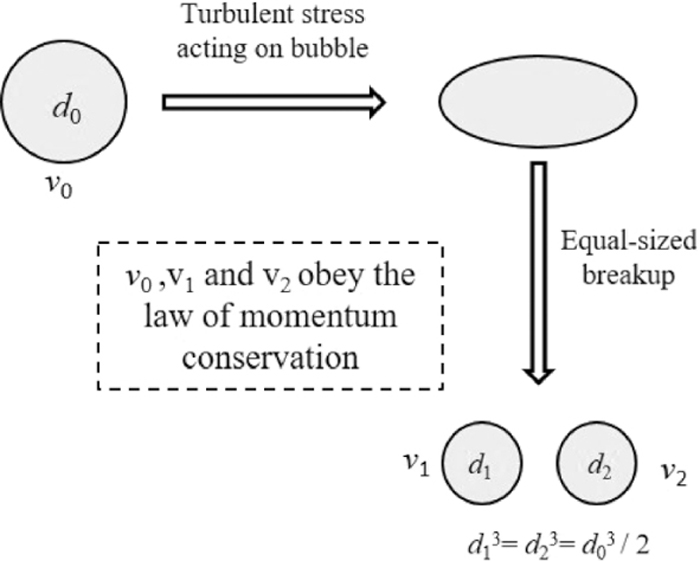Fig. 3.

The schematic diagram of a bubble breakup.Fig. 4.

The flow chart for the model of bubble breakup.

2.2. Fluid-phase Models

Mass and momentum conservation for an incompressible fluid are given by

 ∂ ∂t ( α l ρ l ) +∇⋅( α l ρ l v → l ) =0 (7)

 ∂ ∂t ( α l ρ l v → l ) + u → l ⋅∇( α l ρ l v → l ) =-∇p+∇⋅[ α l ( μ l + μ t ) ]∇ v → l + F ⇀ k (8)
where αl is the liquid-phase volume fraction, ρl is the liquid-phase density, v l is the fluid-phase average velocity, p is pressure, F k is interaction momentum per unit mass transferred from the discrete phases, μl is the liquid viscosity. F k in Eq. (8) is the source term for momentum exchange with the bubbles, representing the drag force, the buoyancy force and virtual mass force respectively.

The liquid-phase volume fraction, αl is defined as

 α l =1- ∑ i V d,i V cell (9)
where, Vd,i is the volume occupied by the discrete phases and Vcell is the volume of the grid cell.

μt in Eq. (4) is the turbulent viscosity, which is defined as

 μ t = C μ ρ l k 2 ε (10)

The standard k-ε model is used to model turbulence, which means that the following transport equations of k and ε are solved.

 α l ρ l ( ∂k ∂t + u → l ⋅∇k ) =-∇( α l μ l σ k ∇k ) + α l G k - α l ρ l ε (11)

 α l ρ l ( ∂ε ∂t + u → l ⋅∇ε ) =-∇( α l μ l σ ε ∇ε )           + α l C 1 ε k G k - α l C 2 ρ l ε 2 k (12)

 G k = μ t ( ∂ u i,j ∂ x j + ∂ u i,j ∂ x i ) ∂ u i,j ∂ x j (13)

The values of the constants are Cμ=0.09, σk=1.0, σε=1.3, C1=1.44, C2=1.92.

2.3. Particle Transport Model

The transient transport of bubbles is simulated based on the flow fields in the liquid pool. The bubbles are treated as discrete phases and their motion can be simulated by integrating the following transport equation for each particle, which considers contributions from six different forces:

 m d d v → d dt = F → d + F → p + F → b + F → vm + F → g + F → L (14)

The terms on the right hand side of Eq. (14) are drag force, pressure gradient force, buoyancy force, virtual mass force, gravitational force, and lift force. All forces in the Eq. (14) are expressed as follows, and details can be seen in previous work.19)

 F → d = C d ρ f | v → - v → d |( v → - v → d ) 2 π d d 2 4 (15)

 F → p = 1 6 π d d 3 ρ l d v → dt (16)

 F → b =- 1 6 π d d 3 ρ l ⋅ g → (17)

 F → vm = 1 6 π d d 3 C vm ρ f d dt ( v → - v → d ) (18)

 F → g = 1 6 π d d 3 ρ g ⋅ g → (19)

 F → L =- 1 6 π d d 3 C L ρ l ( v → d - v → ) ( ∇× v → ) (20)

2.4. Simulation Details

Figure 5 shows the schematic diagram of the mold and grid of the numerical model. In this paper, the three dimensional calculation area was meshed by dividing some domains to reduce the false diffusion in the process of numerical simulation and grid refinement was apply to the submerged entry nozzle to improve the accuracy of the simulation.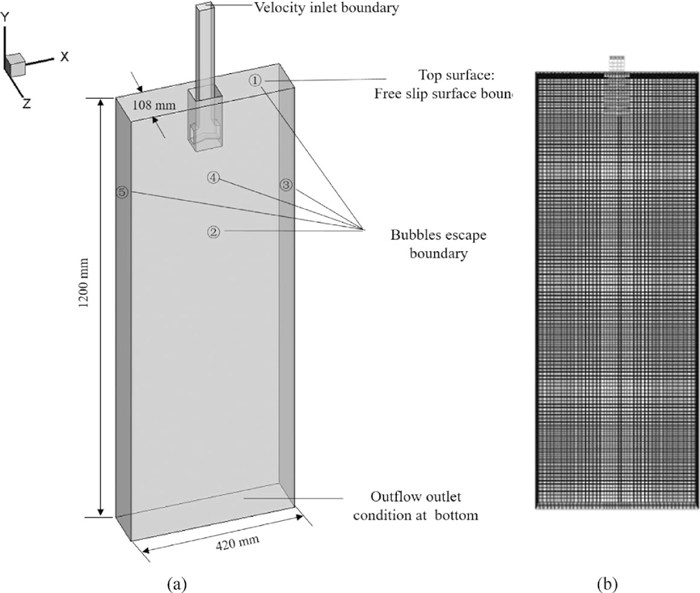Fig. 5.

The schematic diagram of the mold and grid of the numerical model.

The 3D turbulence flow of the fluid and the trajectories of gas bubbles in a continuous casting mold were simulated. Gas bubbles were injected from the wall of the SEN, following the flow stream through the two nozzle ports into the mold. The geometrical and process parameters are given as Table 1.5) The whole grid consists of 150000 cells. The time step for the simulation is 0.001 s.

Table 1. Geometrical and process parameters.
ParametersValue
Slab width (mm)420
Slab thickness (mm)108
Slab length (mm)1200
SEN submerged depth (mm)78, 96, 108
Nozzle exit area (mm2)711
Casting speed (m/min)1.5, 1.9, 2.1
Fluid dynamic viscosity (N·s/m2)0.00555
Fluid density (kg/m3)7020
Gravity acceleration (m/s2)9.81
Gas density (kg/m3)0.291
Gas bubble radius (mm)0.5
Gas volume flow (m3·h−1)0.037, 0.074, 0.101
SEN port angle (degree)15 (downward)

For the melt, a constant velocity inlet boundary condition at the top of the SEN and an outflow outlet condition at the bottom of the calculation domain are applied. The top surface of the liquid being in contact with the slag is assumed to be flat. Here, a free-slip condition is used. The boundary condition between the melt and the SEN wall is no-slip while the SEN itself is considered to be stationary. Meanwhile, all the side walls of the liquid pool are assumed to be stationary and no-slip as well. An escape boundary condition is defined for the mold top surface and at the walls of mold, bubbles were modeled to be caught, while the walls of SEN were modeled to be reflected.

For bubbles, a constant velocity inlet boundary condition is set at the wall of SEN. It is assumed that the bubbles remain spherical and their shape variations are neglected. The velocity is set as zero. From experimental observation, the size of bubble out of same permeable brick are similar, furthermore it is relatively difficult to obtain the exactly actual initial bubble size distribution. Based on previous works and the convenience of calculation, the initial bubble radius was set 0.5 mm as a constant.

3. Model Validation

3.1. Comparison with Bubbles Distribution

Experiments about the behavior of bubbles in the mold have been operated by many researchers. Through comparing with the previous experimental data,21) our numerical simulation results can be validated.

Once the water and air flow reached a steady state, the pictures of bubble dispersion were taken using a high speed camera with 4500 frames per second.21) The distribution of bubbles of water experiment shown the left of Fig. 6, which the operating condition are the water flow rate of 16 L/min, and gas flow rate of 0.833 L/min. Using the mathematical model considering the interaction of bubbles to calculate the distribution of bubbles under the same condition. As shown in Fig. 6, it can be seen that the entire distribution of bubbles in the mold agrees well with the experimental results. Meanwhile the results of mathematical simulation and water experiment reflected the same rule of bubbles motion in the mold. Most of bubbles float up to surface around the SEN in the upper recirculation area, and some smaller bubble flow to further location. Through the image processing software, the mean air bubble diameter of air bubble in water experiment is 1.49 mm. In the results of simulation, the largest diameter of bubble is 3.05 mm, while the smallest diameter is 0.41 mm. And the mean air bubble diameter is 1.63 mm that is similar with the results of water experiment. In conclusion, regardless of the bubbles distribution, motion rule, or mean bubble diameter of the mathematical simulation results agree well with the experimental results.Fig. 6.

The distribution of bubbles in water model and in the calculation model.

3.2. Comparison with Bubbles Interaction

The simplified water model was established to observe the phenomenon of bubbles interaction. The gas bubbles were introduced through brick with porous plug. The movement of bubbles was recorded by a high speed camera with 600 frames per second.

From the Fig. 7, two bubbles in the fluid, the coalescence behavior may occur at any time. Observing three groups of bubbles motion, it found that coalescence phenomenon has nothing with the diameter size of bubbles. As shown in Table 2, bubbles radius of group A and group B are two similar size, but two bubbles of group C are different size. After coalescence, the sum of the volume of two bubbles is equal to the volume of the larger bubble. It’s clear that bubbles coalescence abide by the law of conservation of mass as the description of the mathematical model. Because the phenomenon of a bubble breakup is inverse process of bubbles coalescence, their rule is similar.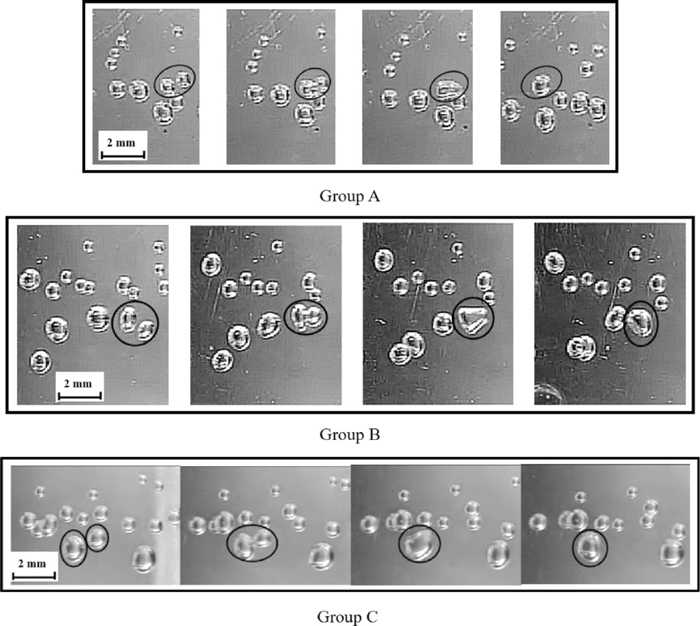Fig. 7.

Three groups of two bubbles coalescence process.

Table 2. Changes of bubble radius and volume before and after coalescence.
GroupsBefore 1Before 2BeforeAfter 3
A1.1400.6481.0780.6362.2182.1610.802
B3.7640.9652.4740.8396.2386.0441.130
C8.3991.2614.1510.99712.55012.3001.432

Figure 8 shows the process of bubbles collision in the water experiment and simulation. From the figure, we can see the whole process of two bubbles collision. When they contact with each other floating in the water, they maybe coalesce into a large bubble.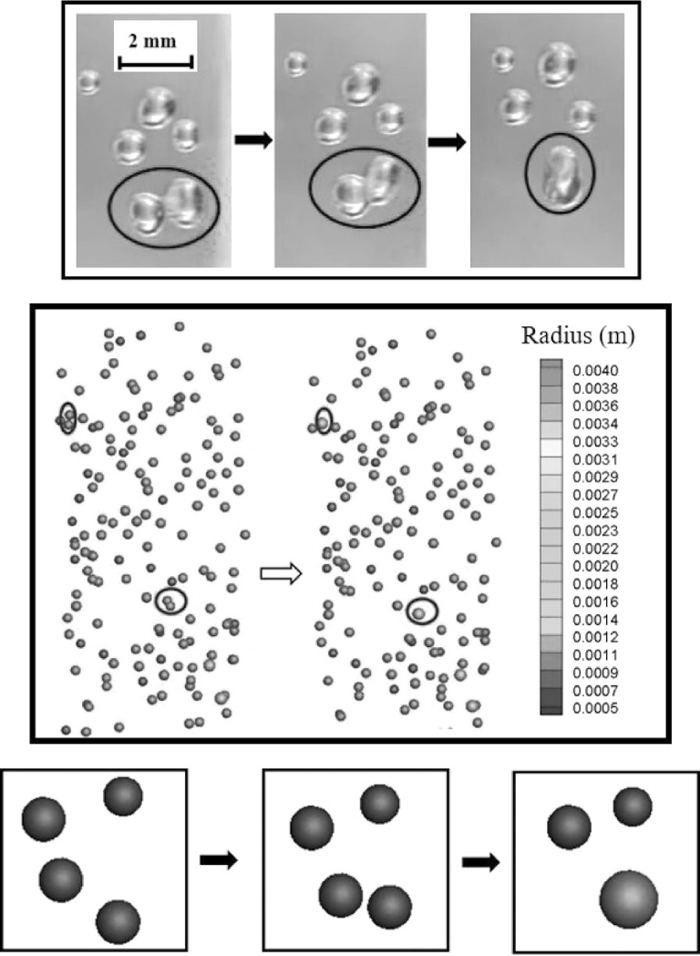Fig. 8.

The process of two bubbles coalesce into a large bubble in the experiment and simulation.

Figure 9 shows the process of a bubble breakup in the water experiment and simulation. From the figure, the phenomenon of a large bubble break into two small bubbles can be observed.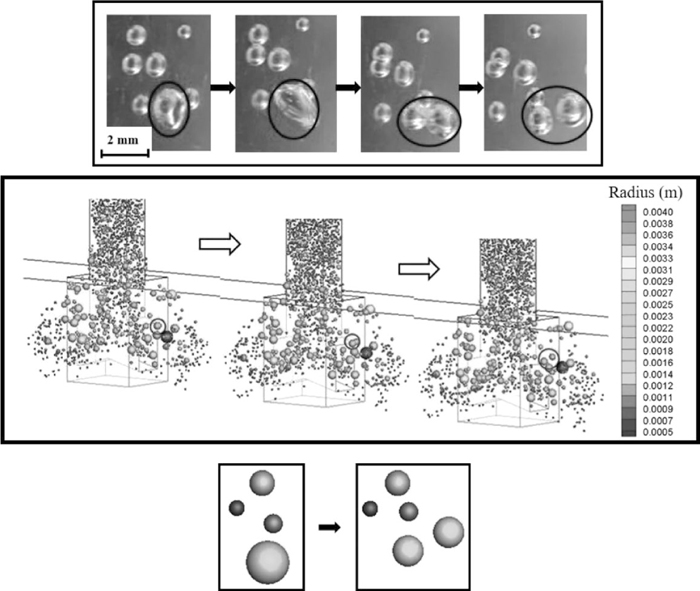Fig. 9.

The process of a bubble break into two small bubbles in the experiment and simulation.

4. Results and Discussions

4.1. Two Phase Velocity Fields

Figure 10 shows the argon bubbles motion, and Fig. 10 shows the gas volume fraction and the velocity at the central plane of the mold with a casting speed of 1.9 m·min−1 and a gas flow rate of 0.074 m3·h−1 and the initial diameter of the argon gas bubble is 1 mm. Bubbles are injected from the top of the SEN into the liquid melt simultaneously. Because of introducing the interaction of bubbles, the size of many bubbles will change after the process of collision and breakup flowing in the melt, and the daughter bubbles size can be directly calculated from the model. Therefore, the results show the motion of different size bubbles in the melt, and in the following discussion we will make a distinction between them. Different color represents different size in the figure.Fig. 10.

The whole distribution of different size bubbles with the submergence depth of 96 mm, the gas flow rate of 0.074 m3·h−1 and casting speed of 1.9 m·min−1.

From the figures, bubbles are diverted into two branches out of ports, and most of them float up to surface in the upper recirculation zone. Then it is rather clear that the larger bubbles rise quickly as they get out of the nozzle side ports, while the others continue to follow the flow to the narrow surface. The smaller bubbles following the flow arrive at the narrow surface, and some of them will be trapped in the solidification front, while most of them will be along with the ascending flow to the top surface and captured by the slag.

From the velocity field of molten steel, in Fig. 11, it can be seen that molten steel from the SEN is divided into two parts forming upper and down recirculation zone. One part flows to the top surface and another part flows to the narrow wall of the mold. And the upper recirculation zone is induced by the argon bubbles. It clear that the velocity is stronger at the position of larger argon volume fraction than other positions; the possible reason is the effect of the bubble buoyancy.Fig. 11.

The velocity vector plot and argon volume fraction distribution in central plane of the mold with the submergence depth of 96 mm, the gas flow rate of 0.074 m3·h−1 and casting speed of 1.9 m·min−1.

Argon gas bubble injection affects the casting process through its influence on the molten steel flow pattern. The extent of this effect depends on the argon gas flow rate, casting speed and the depth of SEN. The huge effects of gas flow rate and bubble size on the flow pattern in the mold have been studied in many previous works. Figures show the effects of these important parameters on the motion of argon bubbles, volume fraction, and radius and number distribution of argon bubbles.

4.2. Effects of the Casting Speed

In the case of the submergence depth of 96 mm, the argon gas flow rate of 0.074 m3·h−1 and the initial bubble radius of 0.5 mm, the distribution of bubbles was simulated with different casting speed. From the figures, the effect of different casting speed on the distribution of bubbles in the mold was observed.

Figure 12 shows the whole distribution of bubbles in the mold with different casting speeds. It can be observed that the rule of distribution is similar under different casting speed. The larger bubbles rise quickly as they get out of the nozzle side ports, while the others continue to follow the flow to the narrow surface. When the casting speed is 1.5 m/min, around the SEN, the radius of bubbles is large but the number of bubbles is less.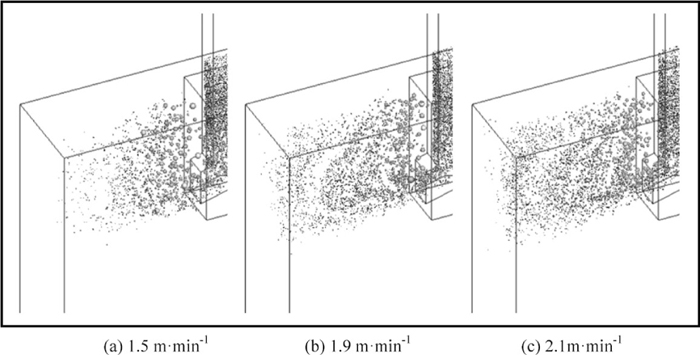Fig. 12.

The whole distribution of argon bubbles with different casting speeds.

When the casting speed increases from the 1.5 to 1.9 and 2.1 m·min−1, the larger shear stress break large bubbles into more small ones. From the figure, the radius of bubbles decreases with the casting speed increasing around the region of 0 to 0.02 m distance from the SEN, but the number of bubbles decreases in this region.

Figure 13 shows the argon volume fraction distribution at the central plane of the mold with different casting speeds. The results illustrate that the range of argon gas volume fraction naturally increases with increasing casting speed. The peak values of argon volume fraction are located at 0.01 m distance from the SEN when the casting speed is 1.5 m·min−1, otherwise, the location is at the region of 0.03 and 0.04 m distance from the SEN when the casting speed is 1.9 m·min−1 and 2.1 m·min−1, respectively.Fig. 13.

The argon volume fraction distribution with different casting speeds.

It can be observed in Figs. 14 and 15 that as the casting speed flow increases, the critical break size of bubbles decreases, so that the process of bubbles break-up is exacerbated out of the SEN which leads to the number of small bubbles increases and the radius of the bubbles decreases, as shown around the region of 0 to 0.02 m distance from the SEN. The float velocity of bubbles with smaller size is higher, thus the gas fraction becomes more reasonable and the upwelling near the nozzle decreases significantly. When the casting speed increases to 1.9 and 2.1 m·min−1, substantial small bubbles move to the narrow side. These small bubbles are easily caught by the solidified shell which might lead to defects in slabs.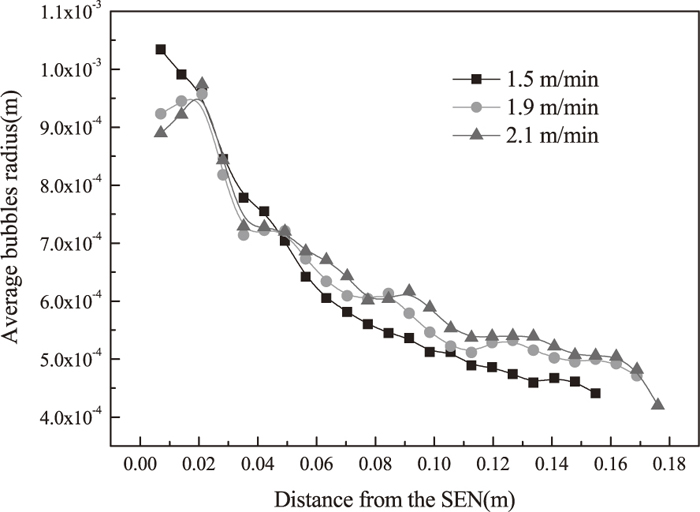Fig. 14.

The size distribution of bubbles with different casting speeds.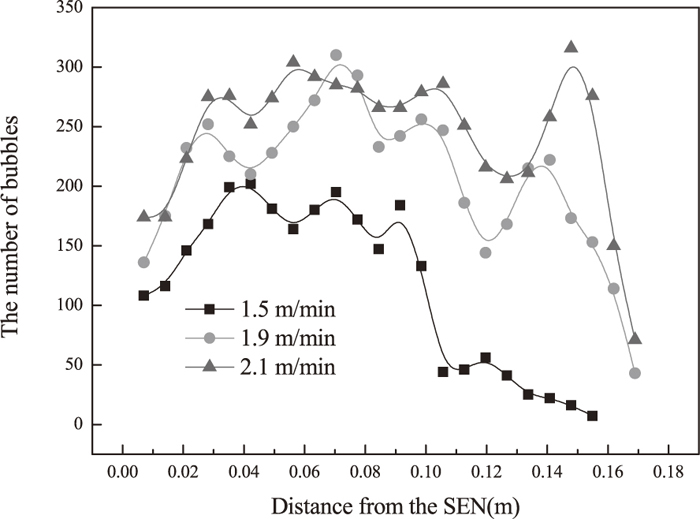Fig. 15.

The quantity distribution of bubbles with different casting speeds.

High casting speed can produce much small bubbles, which is beneficial to promote the efficiency of inclusions removal from the molten steel by argon blowing and improve productivity. However, when the casting speed increases to 2.1 m·min−1, excessive small bubbles move to the narrow side leading to defects in productions. Through comparing the results, 1.5 m·min−1 should be chosen as the casting speed in order to produce high quality products under the condition that immersion depth of nozzle is 96 mm, argon gas flow rate is 0.037 m3·h−1, and the initial radius of bubbles is 0.5 mm.

4.3. Effects of Gas Flow Rate

In the case of the submergence depth of 96 mm, the casting speed of 1.5 m·min−1 and the initial bubble radius of 0.5 mm, the distribution of bubbles was simulated with different gas flow rate. From Fig. 16, the effect of different gas flow rate on the distribution of bubbles in the mold was observed. When the argon gas flow rate increases from 0.037 to 0.101 m3·h−1, the result shows that the radius of bubbles increase and the number of bubbles decreases. And the larger bubbles size will cause slag entrappment when the argon gas flow rate is 0.101 m3·h−1.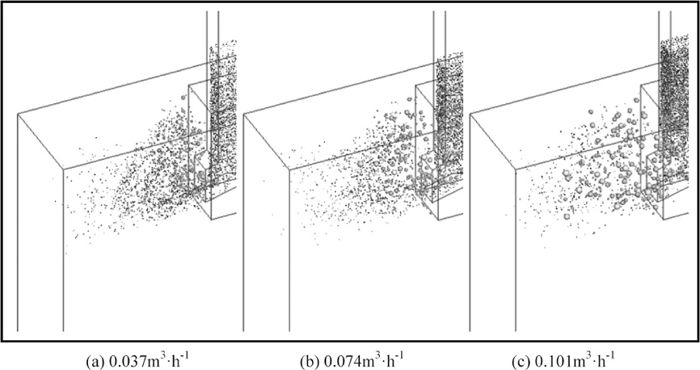Fig. 16.

The whole distribution of argon bubbles with different argon gas flow rates.

On the other hand, Fig. 17 shows the argon volume fraction distribution at the central plane of the mold with different argon gas flow rates. The result illustrates that the argon volume fraction in the mold naturally increases with increasing argon gas flow rate. However, the gas flow rate has nothing effects on the distribution range of argon gas volume fraction. The peak values located at about 0.4 m distance from SEN and most bubbles escape at the region of 0 to 0.12 m distance from the SEN.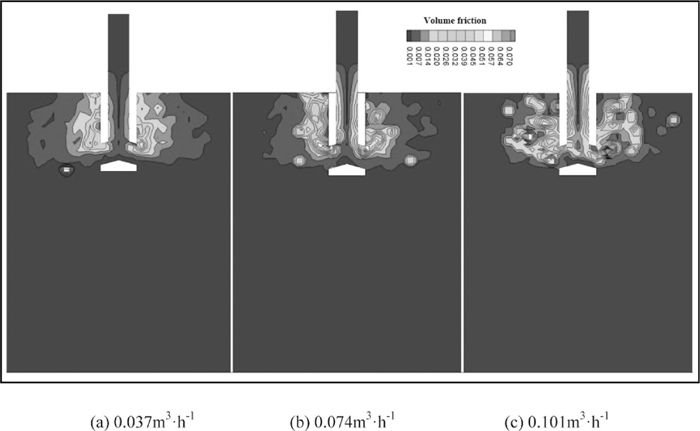Fig. 17.

The argon volume fraction distribution with different argon gas flow rates.

It can be observed in Figs. 18 and 19 that as the argon gas flow rate increases, the process of bubbles coalescence is exacerbated in the SEN and mold which leads to the number of large bubbles increases. Due to the large buoyancy, these bubbles with high velocity float up moving a short distance as soon as get out of the ports. As a result, the gas fraction around the SEN increases significantly and more than other areas, and the upwelling near the nozzle increases obviously. When the gas flow rate increases to 0.101 m3·h−1, substantial large bubbles floating to surface break up around the SEN, causing the slag entrapment.Fig. 18.

The size distribution of bubbles with different argon gas flow rates.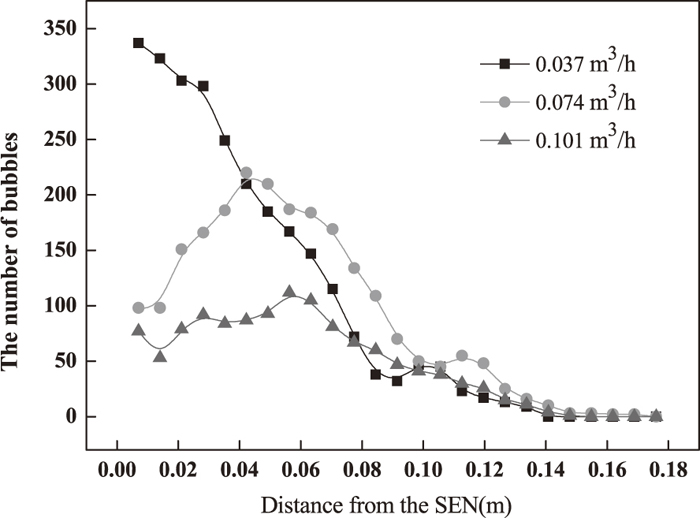Fig. 19.

The quantity distribution of bubbles with different argon gas flow rates.

The injection of more argon bubbles is beneficial to protect SEN against clogging by inclusions. However, excessive gas flow will cause more substantial large bubbles floating to surface break up around the SEN, causing slag entrapment. In conclusion, the optimum gas flow rate is 0.074 m3·h−1 under the condition that immersion depth of nozzle is 108 mm, casting speed is 1.5 m·min−1, and the initial radius of bubbling is 0.5 mm.

4.4. Effects of SEN Depth

In the case of the casting speed of 1.5 m·min−1, the argon gas flow rate of 0.074 m3·h−1 and the initial bubble radius of 0.5 mm, the distribution of bubbles was simulated with different submergence depths. Through this, the effect of different SEN depths on the distribution of bubbles in the mold was observed in Fig. 20. With the SEN depth increasing, as a result, the bubbles have to move a longer distance before floating up to surface from the nozzle, that is, the dispersion of bubbles increases. From Fig. 21, more small bubbles follow the flow to the narrow surface. The results showed that submergence depth has a little effect on the distribution of bubbles size.Fig. 20.

The whole distribution of argon bubbles with different SEN depths.Fig. 21.

The argon gas volume fraction distribution with different SEN depths.

On the other hand, Fig. 21 shows the argon volume fraction distribution at the central plane of the mold with different submergence depths. The result illustrates that the argon volume fraction in the mold naturally increases when the submergence depths increases from 78 mm to 96 mm. However, the argon volume fraction get distributed when the submergence depth is 108 mm. Due to bubbles have more time to flow up to the surface, the distribution range of argon gas volume fraction increases with the submergence depth increasing.

It can be observed from Figs. 22 and 23 that when the submergence depth increase from 78 mm to 96 mm, both distribution of bubbles size and the distribution of bubbles number don’t change obvious. However, when the submergence depth increases to 108 mm, average bubbles size increase slightly and the peak value of bubbles number are located at 0.12 m distance from SEN. All results occurred by reason that bubbles stay in the mold for a longer time with increase of submergence depth, bubbles have more opportunities to get close with others and be collision with others. On the other hand, small bubbles have more time to follow the flow to the narrow wall far away, and these small bubbles are easily caught by the solidified shell which might lead to defects in slabs. But if the submergence depth is too small, it will cause the surface fluctuation. In conclusion, in order to reduce subcutaneous bubbles defects, the better submergence depth is 96 mm under the condition that argon gas flow rate is 0.074 m3·h−1, casting speed is 1.5 m/min, and the initial radius of bubbling is 0.5 mm.Fig. 22.

The size distribution of bubbles with different SEN depths.Fig. 23.

The quantity distribution of bubbles with different SEN depths.

5. Conclusions

In this study, the mathematic model taking the interaction of bubbles into consideration is validated by the experiment results, and with this model, the effects of casting speed, argon gas flow rate and submergence depth on the distribution of bubbles in the mold was investigated. Based on the obtained results, the following conclusions are drawn:

(1)　From the results, good qualitative agreement has been obtained between the simulation results and the experiments about the distribution of bubbles in the mold.

(2)　Bubbles injection can change the flow pattern in the liquid melt. Bubbles are diverted into two branches out of ports, because of introducing the bubbles interaction model, the size of bubbles will change after the process of collision and breakup flowing in the melt. Therefore, it is rather clear that the larger bubbles rise quickly as they get out of the nozzle side ports, while the small others continue to follow the flow to the narrow surface.

(3)　The results illustrate that the range of argon gas volume fraction naturally increases with increasing casting speed. And the number of small bubbles increases and the radius of the bubbles decreases with the increase of casting speed. The argon gas flow rate increases from 0.037 to 0.101 m3·h−1, the result shows that the radius of bubbles increase and the number of bubbles decreases. The argon volume fraction in the mold naturally increases with increasing argon gas flow rate. From the simulation, results show that submergence depth has much impacts on the bubbles distribution range and a little effect on the distribution of bubbles size. The number of bubbles arriving to the narrow wall increases with increasing submergence depth.

(4)　Through comparing the simulation results, the better operate condition is that casting speed is around 1.5 m/min, argon gas flow rate is around 0.074 m3·h−1, and the submergence depth is around 96 mm using this model in order to reduce subcutaneous bubbles defects.

Acknowledgements

The authors are grateful to the National Natural Science Foundation of China for this research (Grant No. 51374081).

Nomenclature

Cd: Drag force coefficient

Cvm: Virtual mass force coefficient

CL: Lift force coefficient

Cμ, C1, C2: Turbulent constant

d: Bubble diameter

fBV: Breakage volume fraction

Fk: Interaction momentum per unit mass transferred from the discrete phase

Fb: Buoyancy force

Fd: Drag force

Fg: Gravitational force

FL: Lift force

Fp: Pressure gradient force

Fvm: Virtual mass force

Gk: Generation of turbulent kinetic energy

g: Acceleration of gravity

m: Bubble mass

p: Static pressure

v d : Relative velocity of two bubbles

v1, v2: Bubble velocity before rebound

v 1 , v 2 : Bubble velocity after rebound

Vd,i: Discrete phase volume

Vcell: Grid cell volume

We: Weber number

Wecrit: Bubble breakup critical Weber number

αl: Liquid volume fraction

σ: Interfacial tension

ρl: Density of fluid phase

ε: Turbulent kinetic energy dissipation rate

μl: Liquid phase viscosity

μt: Turbulent viscosity

References

© 2016 by The Iron and Steel Institute of Japan
Top